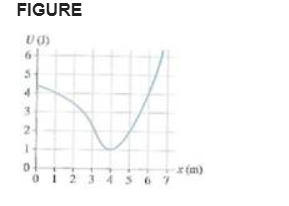# Problem: The figure(Figure 1) is the potential-energy diagram for a 20 g particle that is released from rest at x=1.0m.1. Will the particle move to the right or left?2. How can you tell?3. What is the particle's maximum speed?4. At what position does it have this speed?5. Where are the turning points of the motion?

###### FREE Expert Solution

1.

The particle will move to the right.

2.

ΔK.E = - ΔU

The decrease in potential energy is equal to the gain in kinetic energy.

At x = 1m, the potential energy decreases to the right.

84% (374 ratings)###### Problem Details

The figure(Figure 1) is the potential-energy diagram for a 20 g particle that is released from rest at x=1.0m.1. Will the particle move to the right or left?

2. How can you tell?

3. What is the particle's maximum speed?

4. At what position does it have this speed?

5. Where are the turning points of the motion?